Question

Fluid Mechanics

The figure below shows a piston-cylinder assembly, initially in static equilibrium. Inside the assembly there is pressurized gas with a gauge pressure equal to 150 kPa. If the piston diameter is 0.1 m and the surrounding atmospheric pressure is 100 kPa. Assume that no gas leaks through the interface of the piston and cylinder surfaces and there is no friction between surfaces. Please answer the following.

a. For the initial conditions, the gas and the surroundings have the same temperature. Determine the weight of the piston such that the initial condition of static equilibrium is satisfied. Start your solution by defining a suitable boundary for the gas and showing a free body diagram.Express your answer with a reasonable number of decimal places. In your opinion, could an average person hold the piston on one hand? Why?

b. Next, the gas receives heat and increases its temperature and volume thus lifting the piston by0.3 m while the gas pressure remains constant in a quasi-equilibrium process. Use Eqn. 2-22 of your textbook to determine the work done by the gas to lift the cylinder. Also, sketch the process on a Pressure versus Displacement diagram and on a Pressure Volume diagram. Only use symbols on your axes and associate the initial state with subscript 1 and the final state with subscript 2, i.e., the final state would have a volume V2.

Modify Eqn. 2-22 such that the independent variable is volume. How does it relate to the ketch that you created in part (b)?C.

d. Thinking about potential, kinetic, and internal energy for the gas. Would it change during the process? Explain your reasoning.

e. Assume that from the heat supplied to the air, only 60% gets transformed into work, 20% getstransformed into internal energy, and 20% is transferred to the surroundings. Create anexpression to define the efficiency of this assembly to lift the piston based on the energysupplied.Verified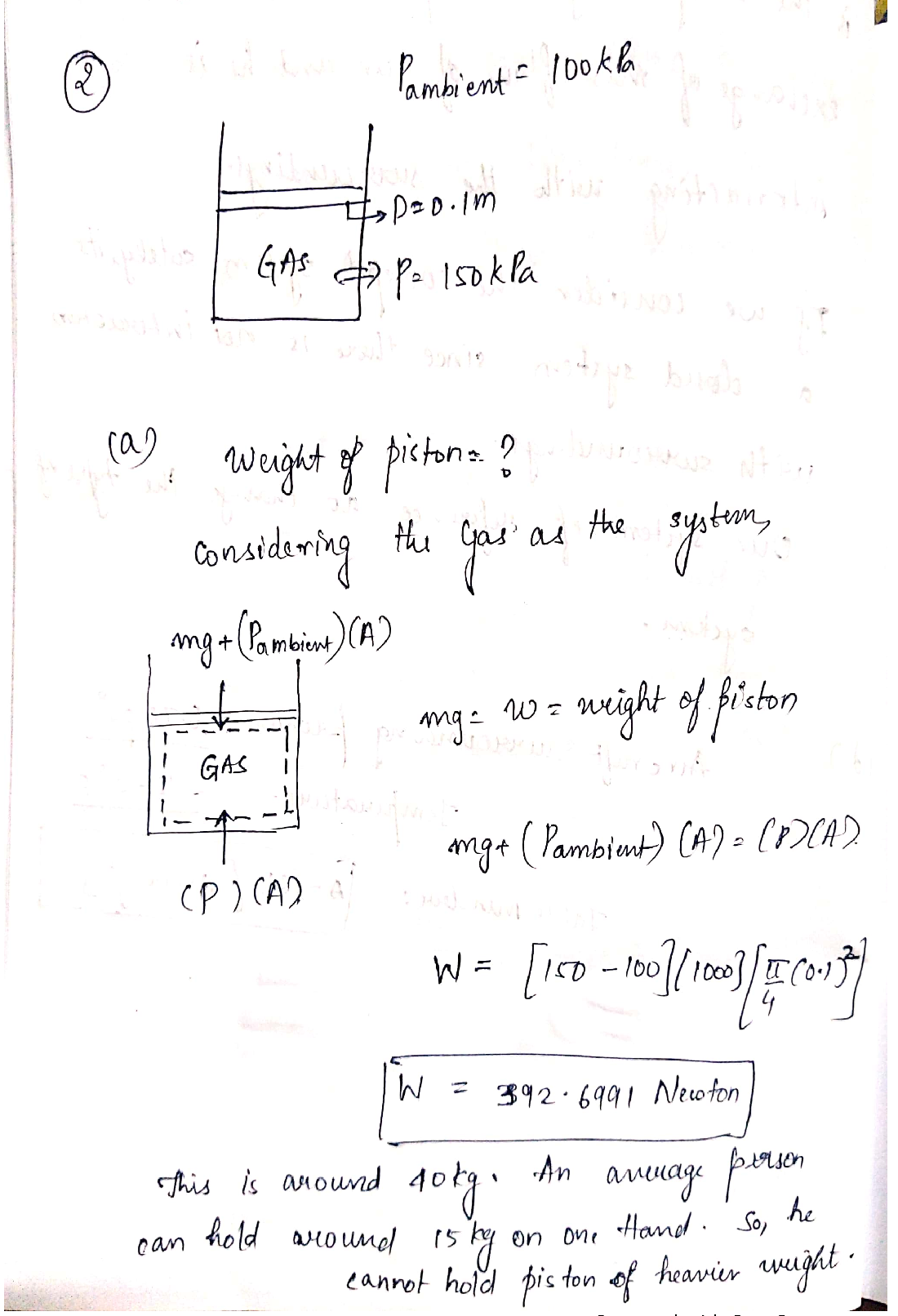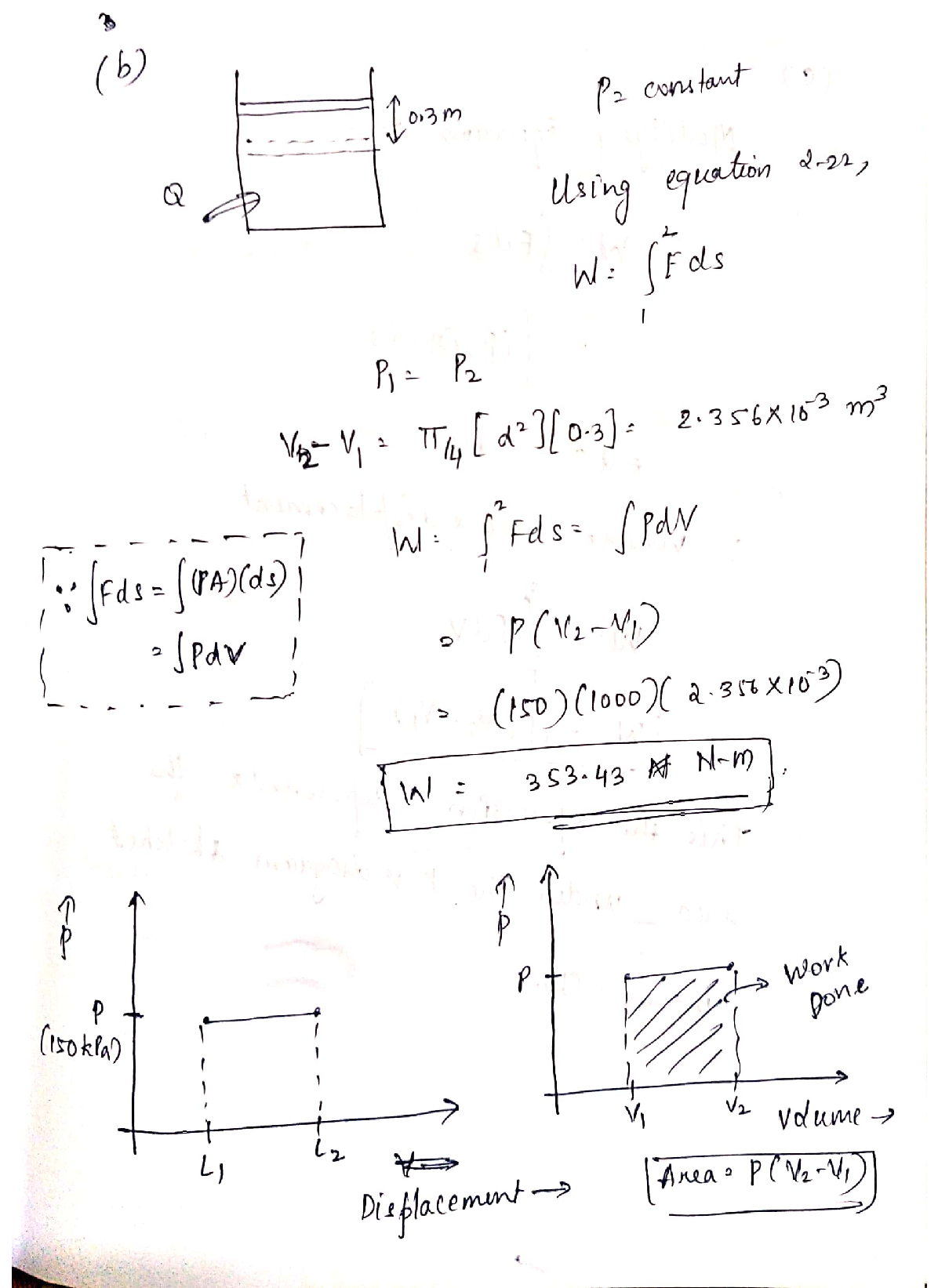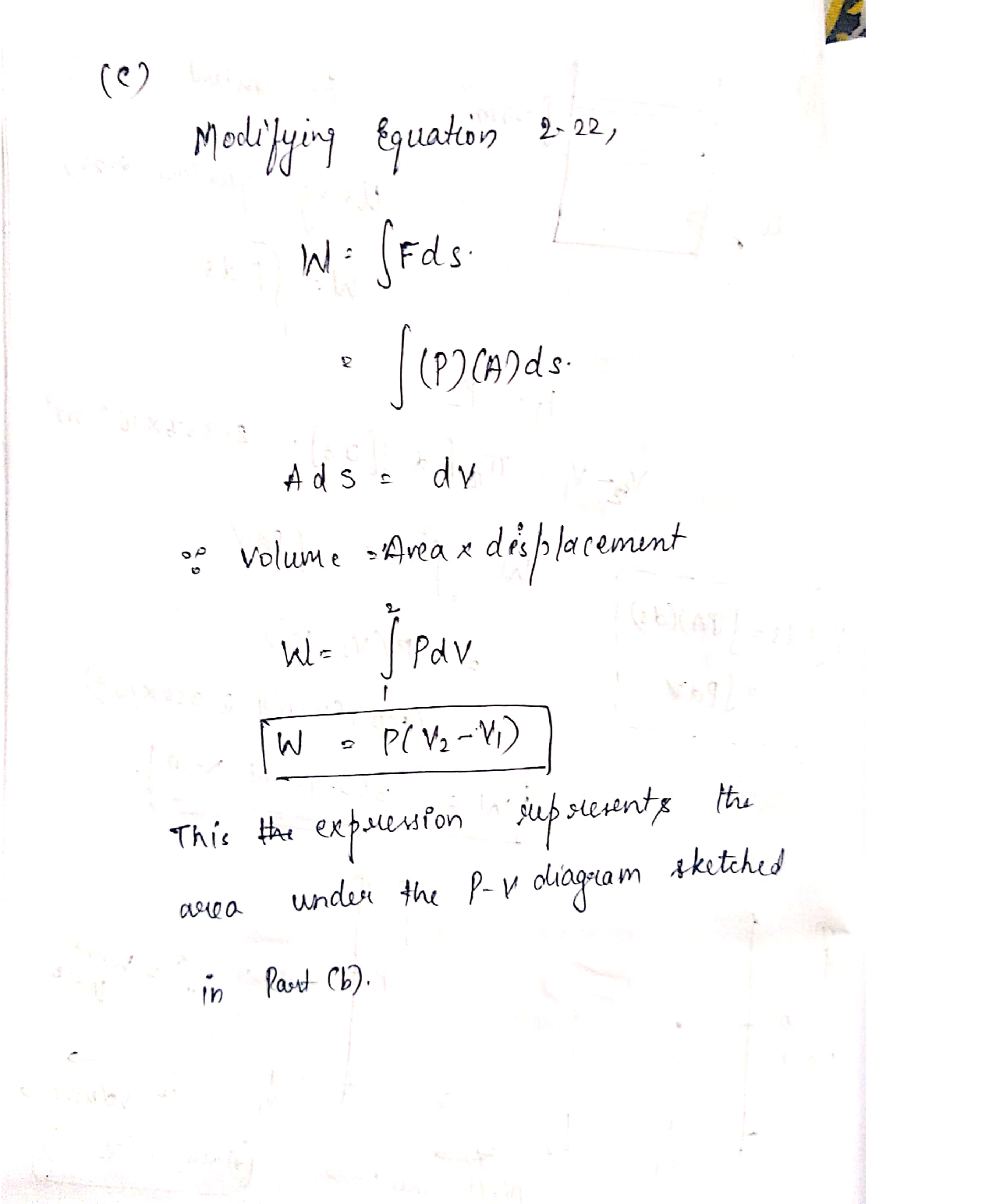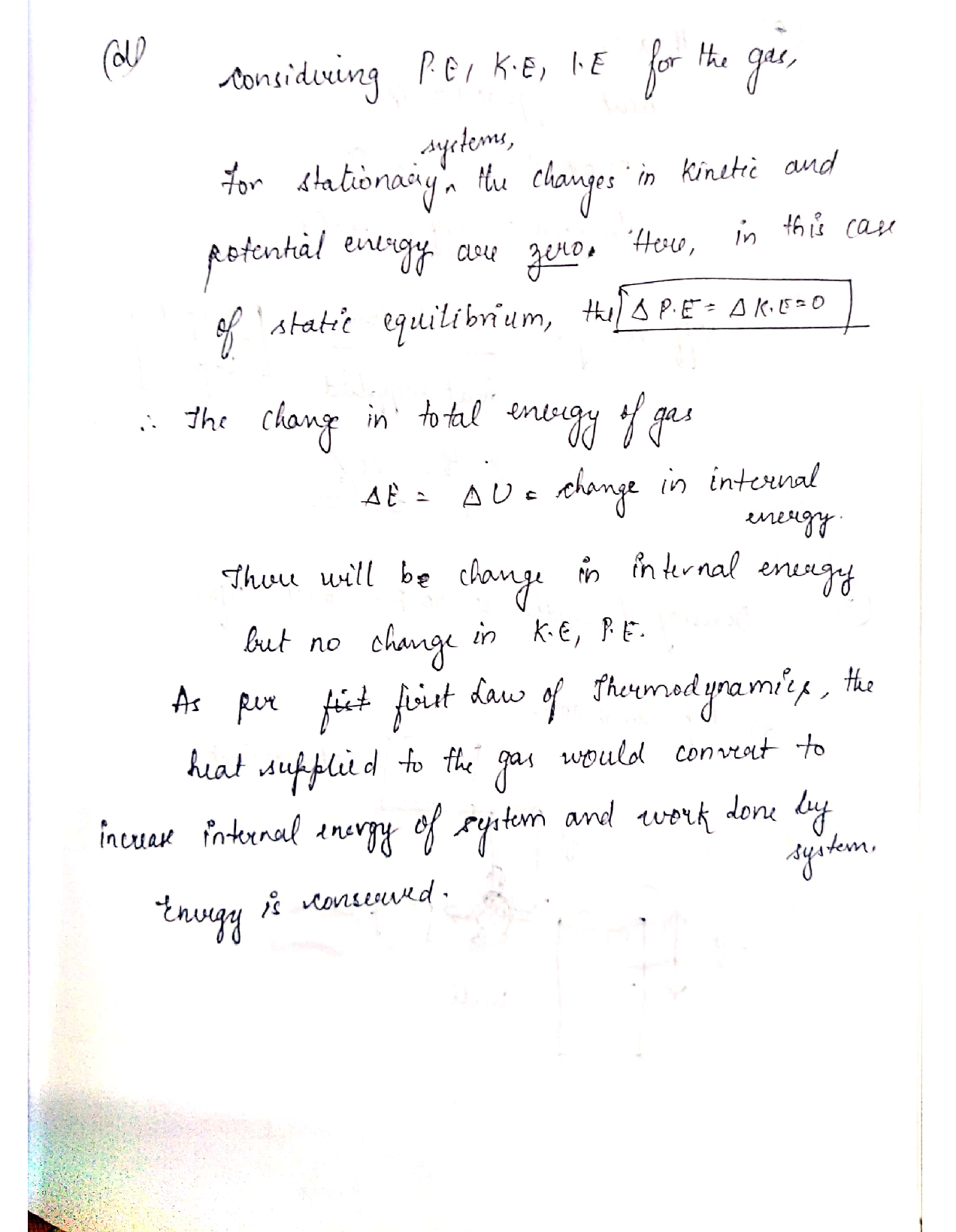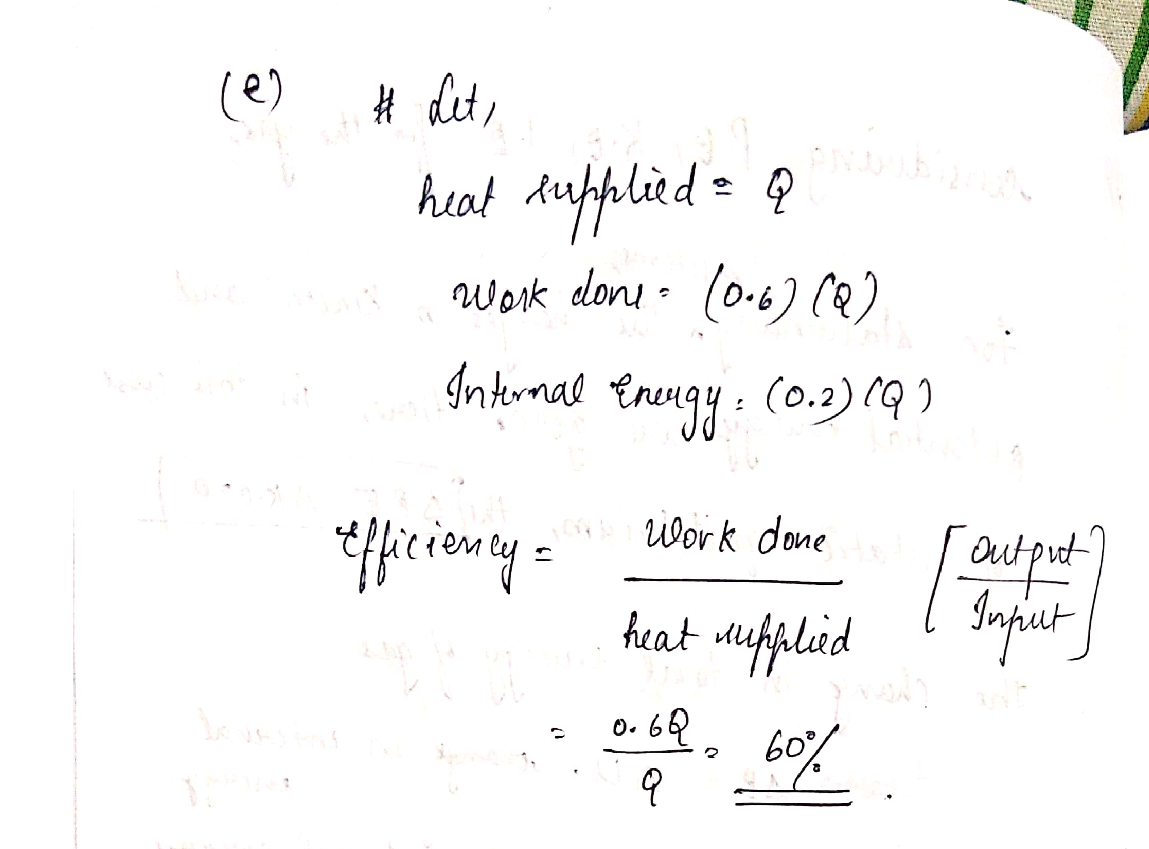### Question 45484Fluid Mechanics

Water in the Belle Fourche Reservoir in Western South Dakotais transported to the water storage reservoir for the town of-Buffalo through a series of three pipes, all with friction factors of 0.02. The first pipe is 500 meters long with a diameter of 0.4meters; the second pipe is 600 meters long with a diameter of0.3 meters; and the third pipe is 500 meters long with a diameter of 0.2 meters. The water surface elevation of the Belle FourcheReservoir is 30 meters higher than the town's storage reservoir.Since the current piping is almost 60 years old, the town of Buffalo has decided to replace the piping system with a single pipe of constant diameter. You have been chosen to calculate the equivalent diameter for the single pipe design and determine the flow rate that will occur. Go to work.

### Question 44899Fluid Mechanics

Transmission lines

### Question 44896Fluid Mechanics

A boat has a volume of 40.0 m³ and a mass of 2.00 tons. What load will push 75.0% of its volume into water? Each Metric ton is 1000 kg.

### Question 44895Fluid Mechanics

A tank has a height of 800 mm and a length of 500 mm. The tank contains a liquid of RD 0.9.Determine:
a. The magnitude of the force on the side of the tank per meter width and its position relative to the base of the tank
b. The force on the base per meter width of tank.

### Question 44894Fluid Mechanics

A closed tank contains three different fluids with a vapor space above the oil, as shown in the figure below. Pressure gauge 1 reads the gauge pressure of the vapor space (P1) and pressure gauge 2 reads the pressure at the bottom of the tank (P2). When P1 = 25 kPa, determine:
a. Height of glycerine (h) in the tank if P2 = 95.66 kPa
. P2 reading if glycerine is replaced with water

### Question 44805Fluid Mechanics

Water flows into cylindrical tank through inlet A and D at rate of 20 ft/s and 5 ft/s respectively. If waterleaves through outlet B and C at rate of 3 ft/s and 10 ft/s respectively.
(A) Derive the continuity equation
\text { Know that } D_{A}=2.5 \text { in, } D_{D}=2 \text { in } D_{B}=3 \text { in and } D_{C}=3.5 \text { in. }
(B) Calculate the change of high h?

### Question 44804Fluid Mechanics

An object which has cubic shape is been floating on the water surface see the diagram below. If the object density is less than water, density find the ratio X/L?

### Question 44803Fluid Mechanics

A force of 15.83 kN is exerted on a piston B (diameter = 250 mm) which fits into a cylinder see the diagram below. How much force is need on piston C to start displacing piston B and Mercury on piston B?

### Question 44802Fluid Mechanics

Given the diagram below if the pressure gauge reads 6.1 psig find Y and X in ft. Given that
\mathrm{SG}_{\mathrm{Aloohal}}=0.9, \mathrm{SG}_{\mathrm{Hg}}=13.6, \text { Alcohol vapor } P=1.7 \mathrm{psig}, Z=4 \mathrm{ft} \text { and } \mathrm{Y}_{\mathrm{H} 2 \mathrm{o}}=62.4 \mathrm{\textrm {lb }} / \mathrm{ft}^{3}

### Question 44801Fluid Mechanics

Given the diagram below, calculate the pressure at A, B, C.
\text { Given that } \mathrm{Y} \text { н2о }=9.79 \mathrm{kN} / \mathrm{m}

### Submit query